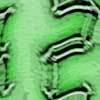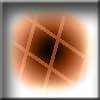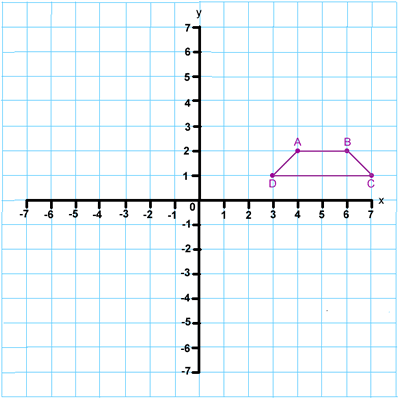#### You may also like### Times

Which times on a digital clock have a line of symmetry? Which look the same upside-down? You might like to try this investigation and find out!### Penta Play

A shape and space game for 2, 3 or 4 players. Be the last person to be able to place a pentomino piece on the playing board.### Clocks

These clocks have been reflected in a mirror. What times do they say?

# Transformation Tease

##### Age 7 to 11Challenge Level

Here is a set of axes with one shape drawn in the first quadrant:What is this shape called? What are the coordinates of the points A, B, C and D which form the corners of the shape?

Translate (or move) the shape 3 squares to the left and 4 squares up. What are its new coordinates?
Compare these with the original coordinates. Do you notice about the numbers?

Start with the shape above again. This time reflect it in the x axis. What are the coordinates of the corners now?

What do you see when you compare these coordinates with the original ones?

Predict what the new coordinates would be after a reflection in the y axis.

Plot these three points on the graph:

(-7, 7)
(0, 0)
(7, -7)

Join the points to make a straight line.
This line is called y = -x because at any point on the line the x coordinate is the same as its y coordinate, but negative.
Reflect the starting shape in the line y = -x and give the new coordinates for A, B, C and D.
Look carefully at these new coordinates and then predict what would happen to these points if they were reflected in the line y = -x:

(2, 4)
(6, -4)
(-5, -5)

Now take the original shape again and rotate it 90 o anticlockwise about the origin. What do you notice about the new coordinates?
How else could you describe this transformation?